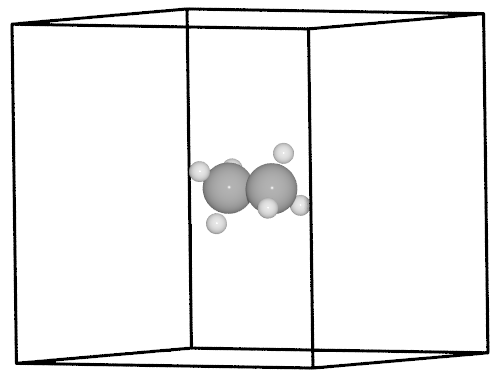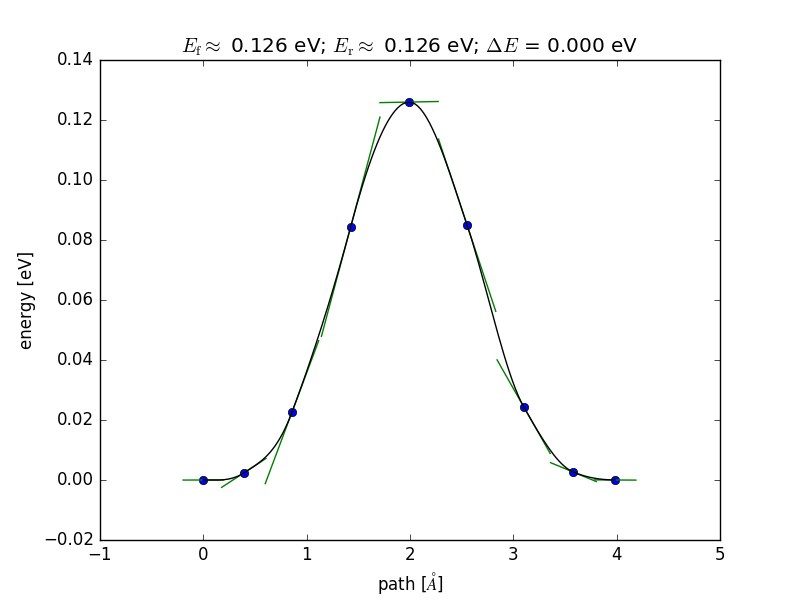# NEBEspresso¶

Derived from the `ase.neb.NEB` class, `NEBEspresso` orchestrates (i)Espresso calculators of individual images in a NEB calculation. This way a `NEBEspresso` object facilitates parallel NEB jobs, where each image calculator uses a subset of the pool of CPUs available to the job.

This tutorial explains the use of `NEBEspresso` to do a NEB calculation on methyl group rotation in ethane, built on this ASE example.

## Initial and final structures of the NEB¶

As opposed to the Effective Medium Potential used in the original example, Quantum Espresso needs periodic boundaries defined by a unit cell. That is defined in the `ethane.traj` trajectory file containing the optimized structure. The final structure is created simply by permuting the H atoms in one of the methyl groups.```from espresso import iEspresso

initial.get_potential_energy()
final = initial.copy()
final.positions[2:5] = initial.positions[[3, 4, 2]]
final.get_potential_energy()
```

## Assigning calculators to intermediate images¶

Initially, images are created as copies of the initial structure and are each asssigned a calculator. Here we use the interactive `iEspresso` calculator, but the non-interactive `Espresso` calculator is equally valid.

```images = [initial]
for _ in range(7):
image = initial.copy()
image.set_calculator(iEspresso(pw=300, dw=4000,kpts='gamma',xc='PBE'))
images.append(image)
images.append(final)
```

## Running the NEB calculation and analyzing the results¶

The `NEBEspresso` class is instantiated with the list of images and is used just as the `ase.neb.NEB` super class, with one exception: the `parallel` keyword. `NEBEspresso` ignores `'parallel'=False` and will always attempt to distribute the image calculators over the CPU pool available to the job.

```from espresso.nebespresso import NEBEspresso
from ase.optimize.fire import FIRE as QuasiNewton

neb = NEBEspresso(images)
neb.interpolate('idpp')

qn = QuasiNewton(neb, logfile='ethane_linear.log', trajectory='neb.traj')
qn.run(fmax=0.05)

from ase.neb import NEBTools
nt = NEBTools(neb.images)
fig = nt.plot_band()
fig.savefig('rotation-barrier.png')
```